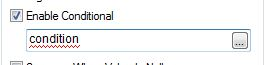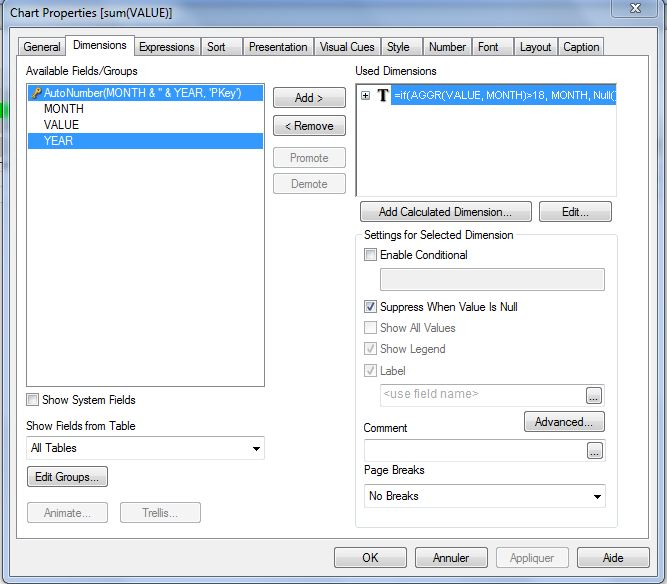# QlikView App Dev

Discussion Board for collaboration related to QlikView App Development.

Announcements
Join us at the Cloud Data and Analytics Tour! REGISTER TODAY
cancel
Showing results for
Search instead for
Did you mean:Contributor III

## Row condition

Hi all,

i this following table and I would like to show only the rows that met this condition : X>b+2*c

How can I do this ?

Thanks

J

4 RepliesNot applicable

If you are only concerned with returning which dimension values meet the above criteria, do the following

In your straight table add the following calculated dimension in the dimension tab

• aggr(if(dimension1>field1+(2*field2),dimension1))
• tick the tickbox that says suppress nulls
• in your expression tab, just add a 1 and then apply

You will be left with a table that shows only dimensions that meet the above criteria. If you want to know exactly what the value was for those met the condition, then instead of 1 in your edit expression, use

• sum(field1)+(2*sum(field2))Not applicable

Hi,

Normally, you should be able to set a condition in the Dimension Tab:Well, it does not work well.

So, Add a calculated dimension and do a conditionnal expression dimensionned by the dimension you want in your report:It should return Null() if the condition is not met, so that you can do "Suppress when value is Null" :Hope this helps

FabricePartner

hi you can change your dimension to calculated dimension

and use this formula

aggr(if(X>b+2*c,object1),object1)Contributor III
Author

hi

This only works if X>b+2*c are not calc fields

X= sum(K)+ sum(W)

b=avg(Aggr(\$(W),Date)

c=sum(K)/sum(W)

been going insane on this

Plz help